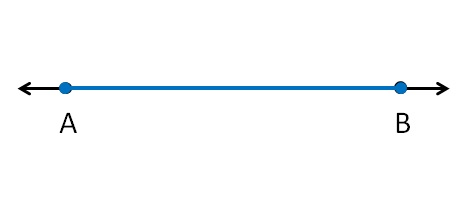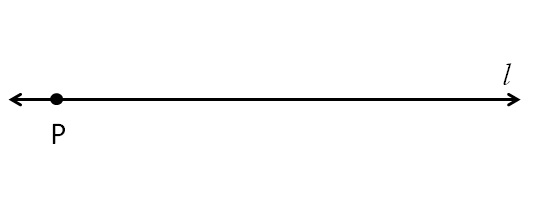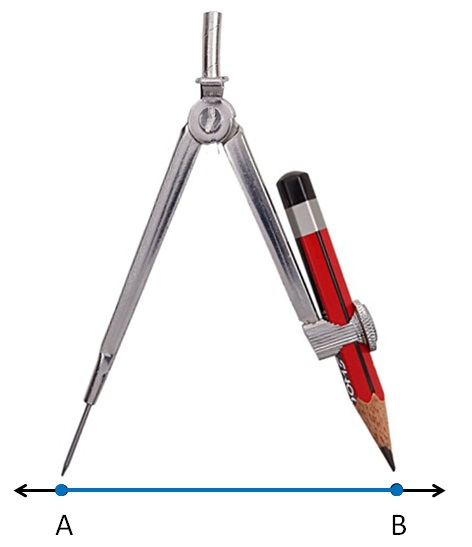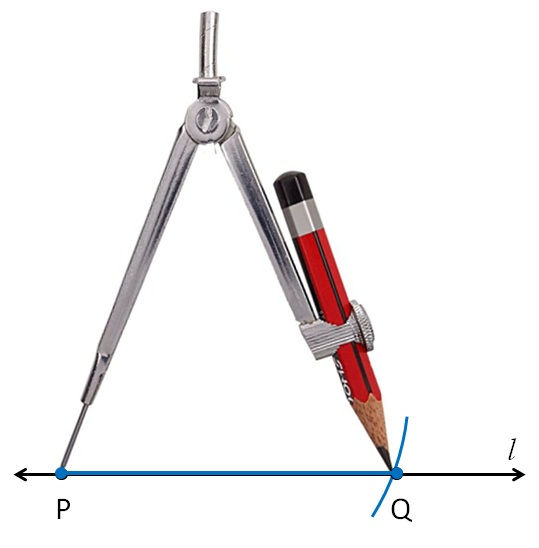Suppose we are given line segment (AB)We need to draw its copy

Draw a line l

mark point P on it1. Measure line segment (AB) using compass3. Now keeping compass opened the same length.

We keep pointed end at point P,

and draw an arc on line lSo, the required line segment is PQ

1. Chapter 14 Class 6 Practical Geometry
2. Concept wise
3. Constructing a copy of a given line segment

Constructing a copy of a given line segment